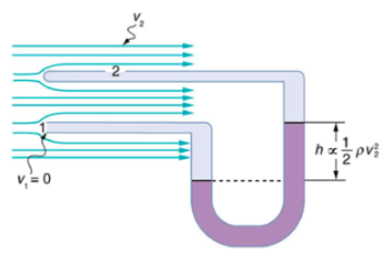# Problem: Suppose you have a pitot tube with a pressure reading at 13 mm Hg at an air speed of 225 km/h. Refer to the figureWhat will the pressure reading be when the wind speed is 345 km/h in mm Hg?

###### FREE Expert Solution

From Bernoulli's theorem, the change in pressure is given by:

$\overline{){\mathbf{∆}}{\mathbf{p}}{\mathbf{=}}\frac{\mathbf{1}}{\mathbf{2}}{\mathbf{\rho }}{{\mathbf{v}}}^{{\mathbf{2}}}}$

80% (73 ratings)###### Problem Details

Suppose you have a pitot tube with a pressure reading at 13 mm Hg at an air speed of 225 km/h. Refer to the figureWhat will the pressure reading be when the wind speed is 345 km/h in mm Hg?